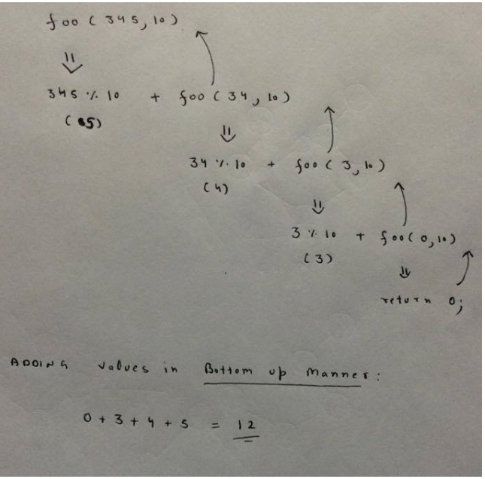# GATE | GATE CS 2011 | Question 48

Consider the following recursive C function that takes two arguments

 `unsigned ``int` `foo(unsigned ``int` `n, unsigned ``int` `r) { ` `  ``if` `(n  > 0) ``return` `(n%r +  foo (n/r, r )); ` `  ``else` `return` `0; ` `} `

What is the return value of the function foo when it is called as foo(345, 10) ?
(A) 345
(B) 12
(C) 5
(D) 3

Explanation: The call foo(345, 10) returns sum of decimal digits (because r is 10) in the number n. Sum of digits for 345 is 3 + 4 + 5 = 12.This solution is contributed by Pranjul Ahuja

Quiz of this Question

My Personal Notes arrow_drop_up
Article Tags :

Be the First to upvote.

Please write to us at contribute@geeksforgeeks.org to report any issue with the above content.